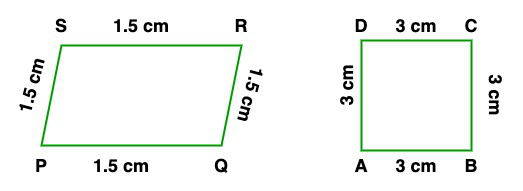GeeksforGeeks App
Open AppBrowser
Continue

# Class 10 NCERT Solutions- Chapter 6 Triangles – Exercise 6.1

### (i) All circles are ……………. . (congruent/similar)

Solution:

All circles are similar because they have radius in fixed proportion.

### (ii) All squares are …………… . (similar/congruent)

Solution:

All squares are similar because the ratio of corresponding sides are equal.

### (iii) All …………….. triangles are similar. (isosceles/equilateral)

Solution:

All Equilateral triangles are similar because the ratio of corresponding sides are equal.

### (iv) Two polygons of the same number of sides are similar, if (a) their corresponding angles are …………… and (b) their corresponding sides are …………… (equal/proportional)

Solution:

Two polygons of the same number of sides are similar, if (a) their corresponding angles are equal and (b) their corresponding sides are proportional.

### (i) similar figures. (ii) non-similar figures.

Solution:

(i) Similar figures

1. All rectangular boards are similar
2. All circular rings are similar

(ii) Non-similar figures

1. A notebook and a pen are non-similar
2. A rectangle and a circle are non-similar.

### Question 3. State whether the following quadrilaterals are similar or not:Solution:

Both the figures are non-similar. For similarity, the corresponding angles should be equal and corresponding sides are in the same ratio. But in this figure ratio of corresponding sides (AB/PQ = AS/PS = DC/SR = CB/RQ = 3/1.5 = 2) but corresponding angles are not equal.

My Personal Notes arrow_drop_up
Related Tutorials